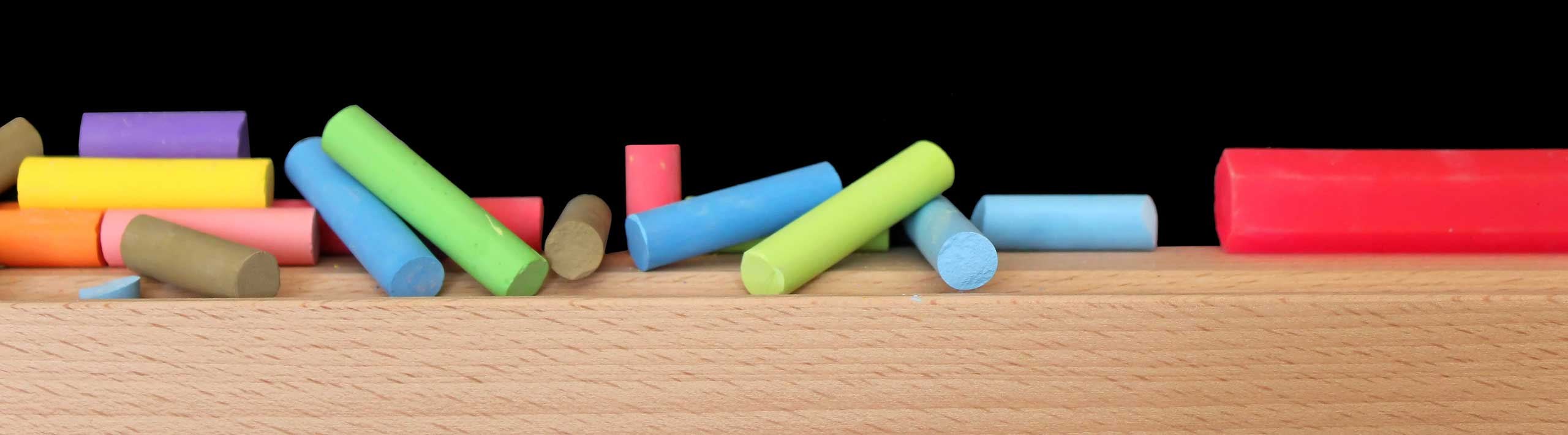# Derivative of Inverse Function

Examples with detailed solutions on how to find the derivative (Differentiation) of an inverse function, in calculus, are presented.

>

## Example 1

Find the derivative dy/dx of the inverse of function f defined by
f(x)= (1/2) x - 1

## Solution to Example 1

We present two methods to answer the above question

### Method 1

• The first method consists in finding the inverse of function f and differentiate it. To find the inverse of f we first write it as an equation
y = (1/2) x - 1
• Solve for x.
x = 2y + 2.
• Change y to x and x to y.
y = 2x + 2.
• The above gives the inverse function of f. Let us find the derivative
dy / dx = 2

### Method 2

• The second method starts with one of the most important properties of inverse functions.
f(f -1(x)) = x
• Let y = f -1(x) so that.
f(y) = x.
• Differentiate both sides using chain rule to the left side.
(dy/dx)(df/dy) = 1.
• Solve for dy/dx
dy / dx = 1 / (df / dy)
• f is defined by
f(x)= (1/2) x - 1
• so that df / dy = 1/2
• Substitute df / dy by 1/2 in dy / dx = 1 / (df / dy) to obtain
dy / dx = 1 / (1/2) = 2
• The first method can be used only if we can find explicitly the inverse function.

## Example 2

Find the derivative dy / dx where y = arcsin x.

## Solution to Example 2

• arcsin x is the inverse function of sin x and
sin(arcsin(x)) = x
• y = arcsin x so that
sin y = x
• Differentiate both sides of the above equation, with respect to x, using the chain rule on the left side.
dy/dx cos y = 1
• Solve for dy/dx.
dy/dx = 1 / cos y
= 1 / cos ( arcsin x)
= 1 / √(1 - sin 2(arcsin x))
= 1 / √ (1 - x 2)

## Exercises

Find the derivative of the inverse of each function.
1) f(x) = 3x - 4
2) g(x) = arccos x
3) h(x) = arctan x

## solutions to the above exercises

1) f -1 ' (x) = 1 / 3
2) g
-1 ' (x) = -1 / √(1 - x 2)
3) h
-1 ' (x) = 1 / (1 + x 2)